# The VLOOKUP Function in Excel

VLOOKUP is an Excel Function that’s used to look up something. It takes four arguments. The first argument is the Something. The second and third arguments are the Look Up part. And the last argument is optional, but the most important, and is either TRUE or FALSE.

Technically the first argument is the lookup_value, but it’s just the Something we’re going to use in the Look Up part. The second and third arguments are the table that holds the look up data and column where the return value resides.

The VLOOKUP Function takes the Something and tries to find a match in the first column of the table.

Now here’s where the TRUE and FALSE part comes in. If the fourth argument is FALSE there will be an exact match. I use FALSE for virtually every VLOOKUP formula, so we’ll consider the TRUE option later.

So to continue, we have the Something lookup_value, and have found an exact match in the first column of the table. This also tells us the particular row of the table where our answer resides.

The third argument tells us the column number of the table to look in. Knowing the row and column gives us the cell where we can find the look up value.

## Using VLOOKUP with FALSE as the Last Argument

So a quick review. We use the VLOOKUP function, where the first argument is something like “Banana.” The second argument tells us where the table is that’s holding the lookup data. We match the word “Banana” in the first column of the table, then slide along the same row to the column number that’s given by the third argument and locate the cell holding the lookup value, which is then returned to the cell where we put the VLOOKUP formula. And of course all of this is guided by the fact that the last argument is FALSE, which tells the VLOOKUP function to get an exact match to “Banana.” (Upper and lower case are considered equivalent.)

Let’s look at a simple example. I have a worksheet named “MyData” that has a table with values we want to look up, shown below.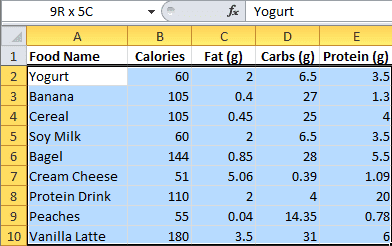Here’s a different worksheet with the VLOOKUP formula.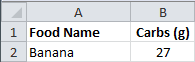Cell B2 holds the VLOOKUP formula:

=VLOOKUP(A2,MyData!\$A\$2:\$E\$10,4,FALSE)

The first argument is cell A2, which is “Banana.”  The second argument is the location of the data table on the MyData worksheet in the range A2:E10. The third argument is the number 4, because we want to look up Carbs (g) in the fourth column, and the last argument is FALSE to find an exact match.

Here’s the VLOOKUP dialog box that you can use in creating the formula. The picture rotates through the four different arguments — Lookup-Value, Table_array, Col_index_num, Range_lookup — so you can see the explanation for each.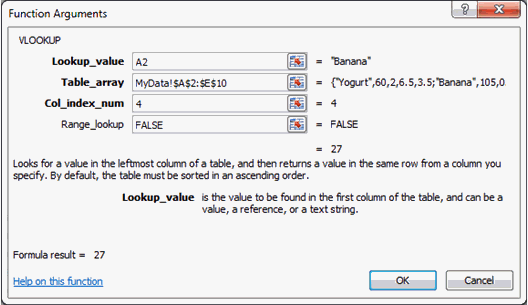One thing you need to know about using FALSE for the fourth argument is that, if there’s more than one of the Something, the VLOOKUP formula will only find the first one in the list.

## Using VLOOKUP with TRUE as the Last Argument

Here’s what you need to know about using TRUE as the last argument.

1. The values in the first column must be placed in ascending order, otherwise you might not get the correct value.
2. If you omit the last argument, VLOOKUP considers it to be TRUE.
3. With TRUE, you get an exact or approximate match. If no exact match is found, then you get the next largest value that’s less than the lookup_value. (And after all these years, this still strains my brain to near the breaking point.)

Here’s a simple example that shows how grades are returned by a VLOOKUP formula when given some numerical scores.  The data table for grades is shown here.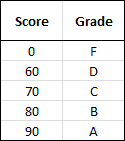• Any score with values from 0 – 59 will return an F grade.
• Any score with values 60 – 69 will return a D grade.
• Any score with values 70 – 79 will return a C grade.
• Any score with values 80 – 89 will return a B grade.
• Any score with values 90 – 100 will return an A grade.

I covered this in an earlier article: Grade Formulas in Excel.

Here’s the worksheet with Students, Scores, and the VLOOKUP formula.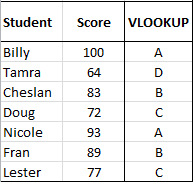And here’s the VLOOKUP formula used with TRUE as the fourth argument.

The first argument is the scores in the Score column, which I gave the name: Scores. The second argument is the table that I’ve named GradeLU. The third argument is 2 because the second column has the Grades.

If the first argument is 83, the VLOOKUP formula looks to the GradeLU table, and since the last argument is TRUE, it looks in the first column and matches the 80, then goes to the second column and returns the grade B.## 1 thought on “The VLOOKUP Function in Excel”

1.Dennis Taylor

Instead of using FALSE for an exact match, you can use 0 (zero) instead.
It’s less typing and it’s more in sync with the MATCH function, which uses zero for an exact match. Yes, I know, you can use FALSE instead of zero in the MATCH function.

The word FALSE, even though it works just fine, seems to suggest that something failed, or didn’t match up properly. Perhaps the programmers could have used the word EXACT, but the long-term usage of TRUE and FALSE in programming environments is not about to be dislodged.